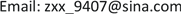1. 引言

2. 方法2.1. 记号

2.2. 方法

c l r ( X ) = ( log X 1 g ( X ) , ⋯ , log X p g ( X ) ) (1)

g ( X ) = ( ∏ i = 1 p X i ) 1 / p 是X的几何均值。

S i = c l r (Xi)

γ j k = cov ( S j , S k ) (2)

f i ( Γ ^ , B ) = 1 2 β i T Γ ^ β i − β i T e i (3)

Γ ^ 是正定的，则 f i 是凸函数，当损失函数趋于0时，损失函数越小，B越趋近 Ω 。

1 2 β T Γ ^ β − e i T β + λ n i | β | 1 (4)

β ^ i = arg min β ∈ R p { 1 2 β T Γ ^ β − e i T β + λ n i | β | 1 } (5)

λ ^ i = arg min 0 ≤ j ≤ N { 1 H ∑ v = 1 H [ 1 2 ( β ^ i − v ( λ j ) ) T Γ ^ v β ^ i − v ( λ j ) − e i T β ^ i − v ( λ j ) ] } (6)

Ω ^ = ( ω ^ i j ) p × p 其中 ω ^ i j = ω ^ j i = β ^ i j I { | β ^ i j | < | β ^ j i | } + β ^ j i I { | β ^ i j | ≥ | β ^ j i | } 。

3. 数值模拟

W k j = e Y k j ， X k j = W k j / ∑ i = 1 p W k i , j = 1 , ⋯ , p (7)

The precision matrix performance index under different transformations obtained in mode
pΩ ^Ω ^ YΩ ^ l

500.74640.74403.0591
1000.55690.55502.4696
2000.45540.45422.1795
Frobenius范数
502.45772.424510.8431
1002.90032.874513.7074
2003.78983.771318.6849

The precision matrix performance index under different transformations obtained in mode
pΩ ^Ω ^ YΩ ^ l

500.12120.11870.5845
1000.11900.11770.6227
2000.11950.11880.6112
Frobenius范数
500.62860.61602.9426
1000.85740.84864.0881
2001.18971.18385.7714

4. 与炎症性肠病(IBD)相关的细菌物种数据集分析

IBD数据集收集了85例IBD病例的粪便样本和26个正常对照样本，并对每个样品进行宏基因组测序，从而鉴定出总共97种细菌物种  。对于数据集中的零元素，在不超过数据生成过程中的最小探测精度的条件下，我们取103。取正常样本( k = 1 )的1/5，病例样本( k = 2 )的1/5组成测试集，其他样本组成训练集。然后我们对数据集进行线性判别分析，其分析模型可以参见文献  。

δ k ( X ) = X T Ω ^ μ ^ k − 1 2 μ ^ k T Ω ^ μ ^ k + log π ^ k (8)

T P R = T P T P + F N ， F P R = F P F P + T N (9)

M C C = T P × T N − F P × F N ( T P + F P ) ( T P + F N ) ( T N + F P ) ( T N + F N ) (10)

Classification performance resul
TPRFPRMCC
0.85710.04550.8117

5. 结语